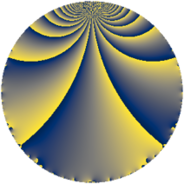Properties

 Label 105.2.dLevel $105$ Weight $2$ Character orbit 105.d Rep. character $\chi_{105}(64,\cdot)$ Character field $\Q$ Dimension $8$ Newform subspaces $2$ Sturm bound $32$ Trace bound $1$

Related objects

Defining parameters

 Level: $$N$$ $$=$$ $$105 = 3 \cdot 5 \cdot 7$$ Weight: $$k$$ $$=$$ $$2$$ Character orbit: $$[\chi]$$ $$=$$ 105.d (of order $$2$$ and degree $$1$$) Character conductor: $$\operatorname{cond}(\chi)$$ $$=$$ $$5$$ Character field: $$\Q$$ Newform subspaces: $$2$$ Sturm bound: $$32$$ Trace bound: $$1$$ Distinguishing $$T_p$$: $$2$$

Dimensions

The following table gives the dimensions of various subspaces of $$M_{2}(105, [\chi])$$.

Total New Old
Modular forms 20 8 12
Cusp forms 12 8 4
Eisenstein series 8 0 8

Trace form

 $$8 q - 8 q^{4} + 4 q^{5} - 4 q^{6} - 8 q^{9} + O(q^{10})$$ $$8 q - 8 q^{4} + 4 q^{5} - 4 q^{6} - 8 q^{9} - 8 q^{10} + 4 q^{15} + 24 q^{16} - 28 q^{20} + 4 q^{21} + 12 q^{24} - 8 q^{25} + 24 q^{26} - 12 q^{30} - 16 q^{31} + 32 q^{34} + 4 q^{35} + 8 q^{36} - 8 q^{39} + 16 q^{40} + 8 q^{41} - 32 q^{44} - 4 q^{45} - 16 q^{46} - 8 q^{49} - 8 q^{50} + 8 q^{51} + 4 q^{54} - 8 q^{55} - 24 q^{56} - 16 q^{59} - 4 q^{60} - 16 q^{61} - 40 q^{64} + 24 q^{65} + 8 q^{66} + 8 q^{69} + 8 q^{70} + 32 q^{71} + 80 q^{74} + 16 q^{75} + 16 q^{76} - 32 q^{79} + 44 q^{80} + 8 q^{81} - 12 q^{84} + 16 q^{85} - 40 q^{89} + 8 q^{90} + 16 q^{91} + 32 q^{94} + 16 q^{95} - 68 q^{96} + O(q^{100})$$

Decomposition of $$S_{2}^{\mathrm{new}}(105, [\chi])$$ into newform subspaces

Label Dim. $$A$$ Field CM Traces $q$-expansion
$a_{2}$ $a_{3}$ $a_{5}$ $a_{7}$
105.2.d.a $2$ $0.838$ $$\Q(\sqrt{-1})$$ None $$0$$ $$0$$ $$2$$ $$0$$ $$q+iq^{2}+iq^{3}+q^{4}+(1-2i)q^{5}-q^{6}+\cdots$$
105.2.d.b $6$ $0.838$ 6.0.350464.1 None $$0$$ $$0$$ $$2$$ $$0$$ $$q-\beta _{1}q^{2}+\beta _{4}q^{3}+(-1+\beta _{3}+\beta _{5})q^{4}+\cdots$$

Decomposition of $$S_{2}^{\mathrm{old}}(105, [\chi])$$ into lower level spaces

$$S_{2}^{\mathrm{old}}(105, [\chi]) \cong$$ $$S_{2}^{\mathrm{new}}(35, [\chi])$$$$^{\oplus 2}$$